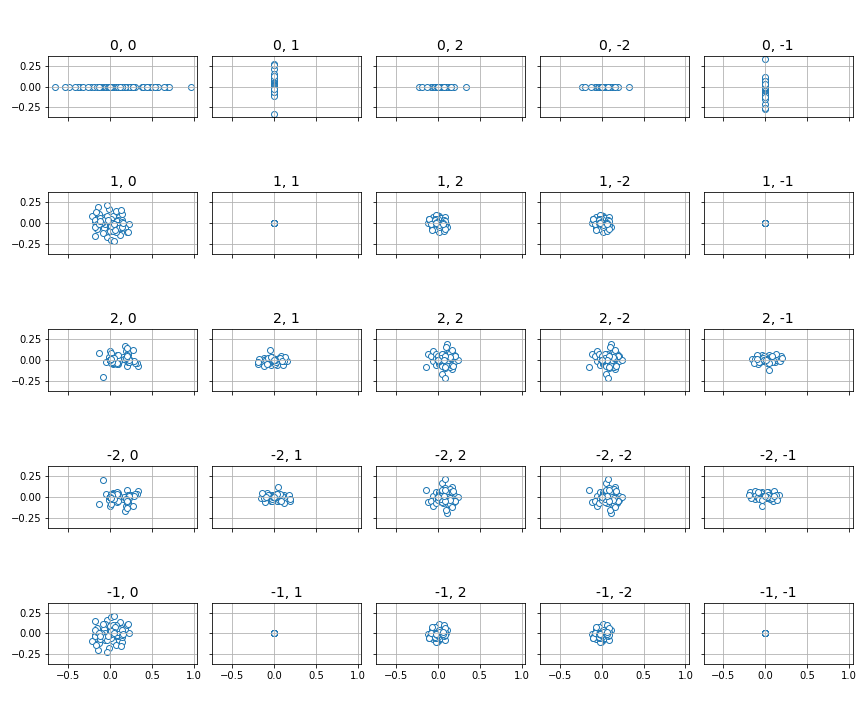# qml.fourier.visualize.panel¶

panel(coeffs, n_inputs, ax, colour=None)[source]

Plot a list of sets of coefficients in the complex plane for a 1- or 2-dimensional function.

Parameters
• coeffs (list[array[complex]]) – A list of sets of Fourier coefficients. The shape of the coefficient arrays must all be either 1- or 2-dimensional, i.e., each array should have shape (2d + 1,) for the 1-dimensional case, or (2d + 1, 2d + 1) where d is the degree, i.e., the maximum frequency of present in the coefficients. Such an array may be the output of the numpy/scipy fft/fft2 functions, or coefficients().

• n_inputs (int) – The number of variables in the function.

• ax (array[matplotlib.axes._subplots.AxesSubplot]) – Axis on which to plot. For 1-dimensional data, length must be the number of frequencies. For 2-dimensional data, must be a grid that matches the dimensions of a single set of coefficients.

• colour (str) – The outline colour of the points on the plot.

Returns

The axes after plotting is complete.

Return type

array[matplotlib.axes.Axes]

Example

Suppose we have the following quantum function:

dev = qml.device('default.qubit', wires=2)

@qml.qnode(dev)
def circuit_with_weights(w, x):
qml.RX(x, wires=0)
qml.RY(x, wires=1)
qml.CNOT(wires=[1, 0])

qml.Rot(*w, wires=0)
qml.Rot(*w, wires=1)
qml.CNOT(wires=[1, 0])

qml.RX(x, wires=0)
qml.RY(x, wires=1)
qml.CNOT(wires=[1, 0])

return qml.expval(qml.PauliZ(0))


We would like to compute and plot the distribution of Fourier coefficients for many random values of the weights w. First, we generate all the coefficients:

from functools import partial

coeffs = []

n_inputs = 2
degree = 2

for _ in range(100):
weights = np.random.normal(0, 1, size=(2, 3))
c = coefficients(partial(circuit_with_weights, weights), n_inputs, degree)
coeffs.append(c)


We can now plot by setting up a pair of matplotlib axes and passing them to the plotting function. The of axes must be large enough to represent all the available coefficients (in this case, since we have 2 variables and use degree 2, we need a 5x5 grid.

>>> import matplotlib.pyplot as plt
>>> fig, ax = plt.subplots(5, 5, figsize=(12, 10), sharex=True, sharey=True)
>>> panel(coeffs, n_inputs, ax)